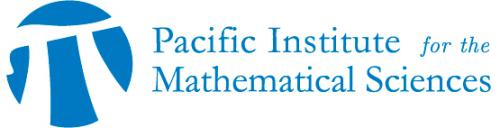## PIMS—UManitoba Distinguished Lecture: Joan Boyar

• Date: 10/18/2018
Speaker(s):

Joan Boyar

(University of Southern Denmark)

Location:

University of Manitoba

Topic:

Multiplicative Complexity of Cryptographic Functions [video]

Description:

A video of this event is available on mathtube.org.

A symmetric key cryptosystem is one in which the same secret key is used for both encryption and decryption. An encryption function in a block symmetric key cryptosystem is a function of both the key and a block of n bits of data, and the result would generally be n bits long. The bits can be considered to be values in GF(2), and these functions are called Boolean functions. Such an encryption function must be highly nonlinear, or the system can be broken.

One measure of the nonlinearity of a Boolean function is its multiplicative complexity, which is the number of modulo 2 multiplications (ANDs) needed to compute the function, if the only operations allowed are multiplication and addition of two values modulo 2 (AND and XOR) and adding 1 modulo 2 to a value (NOT). This talk will be a survey of some results concerning multiplicative complexity, including a comparison to some other measures of nonlinearity. Multiplicative complexity turns out to be interesting in a another way in settings such homomorphic encryption and multi-party cryptographic protocols, where it can be important that the functions being computed have low multiplicative complexity.

Schedule:

When: 4:00pm
Where: Robert B. Schultz Lecture Theatre
Day: October 18, 2019